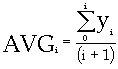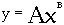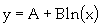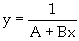# Transforms

Provides several functions to calculate running statistics, regressions, FFTs, etc.

Histograms (abs)
Histograms (rel)
Creates a simplified histogram (plot) representation of selected data, showing the relative frequency that a given value appears in the vector, or its absolute value.
Fourier Transforms
Rectangular
Rectangular window applied to data in the frequency domain.
Hanning
Hanning windowing function.
Welch
Welch windowing function.
Hamming
Hamming windowing function.
Blackman
Blackman windowing function.
Parzen
Parzen windowing function.
Inverse
Matrix inversion function.
Running Stats
Average
The statistical measures are made at the current point, using all prior data points.
Median
MinimumMaximum
Std. Dev.
Regression
For linear through 10th degree: Polynomial least squares curve fit function.
Linear
Cubic
4th-10th degree
Powery = A*xB
Fit given data to, where A and B are calculated for best fit.
Exponentialy = A*exp(B*x)
Exponential least-squares curve fit function.
Logarithmicx = A+B*In(x)
Fit given data to, where A and B are calculated for best fit.
Inversey = I/(A+B*x)
Fit given data to, where A and B are calculated for best fit.
Derivative
Curve derivative function.
Integral
The integral function.
Cross Correlation
Cross correlation between two curves.
Output ➔➔
x: lag
y: correlation (A value of 1 is a perfect correlation)
For example, at a lag of, c1 and c2 have a correlation value of "correlation."
Auto Correlation
Splines
Cubic spline interpolation function.
Sample Points
Resample or sample points. Resamples the given input curve to the specified data frequency.
Digital Filter
Impulse response filter function.